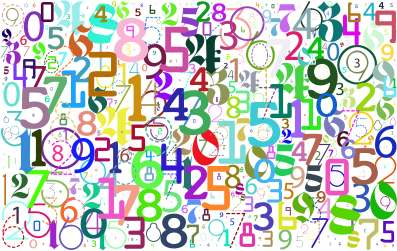# Prime Decomposition

Instructions: Compute the prime decomposition of a non-negative integer value $$n$$. The value of $$n$$ needs to be integer and greater than or equal to 1.The integer $$n$$ =

## How to Use this Prime Decomposition Calculator

More about Prime Decomposition : For an integer number $$n$$, there exists a unique prime decomposition, this is, a way of expressing this integer number $$n$$ as a product of different prime numbers (where those prime numbers can be repeated, or have multiplicity, as it is commonly said as well).

For example, the number $$n = 12$$ can be written as it follows

$12 = 3 \cdot 4$

Is this the prime decomposition of $$n = 12$$? Nope, because 3 is a prime number (it is divisible only by 1 and by itself), but 4 is not prime (because it is divisible by 2). So then, the decomposition shown above is a decomposition, but not the the prime decomposition. Now, observing that

$12 = 3 \cdot 4 = 3 \cdot 2 \cdot 2$

we can see that now $$n = 12$$ is decomposed as the product of primes only. Reordering the primes in ascending order, and grouping the primes with multiplicity, we get the neat expression

$12 = 2^2 \cdot 3$## Application of Prime Decomposition

There are multiple applications of prime decomposition, perhaps the most common ones is its use for getting the greatest common divisor between two numbers and to reduce a fraction to its lowest expression .

This solver with provide you a prime factorization calculation with steps. Check our some more Algebra Calculators from our site, or you can try some external ones### Example: Prime Factorization

Find the prime factorization of $$n = 108$$

Solution: Observe that $$n = 108$$ is even, so then $$2$$ is a factor. So we get $$n = 108 = 2 \cdot 54$$. Now, 54 is also an even number and we know that $$54 = 2 \cdot 27$$. Hence

$n = 108 = 2 \cdot 2 \cdot 27$

But $$27 = 3^3$$, so then we get the following prime factorization:

$n = 108 = 2^2 \cdot 3^3$